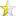Dec 14, 2012 (last update: Jan 5, 2013)

# Caesar cipher### Caveat

The Caesar cipher encrypts text by rotating the alphabet, leaving digits and symbols unchanged. It was used in ancient times to encrypt confidential messages, but from today's perspective it is just a toy cipher.

### Goal

• To provide a means to encrypt `char[]`'s, `std::string`s, and files.
• It is achieved by a function template which works with iterators.

### Language

C++11, the 2011 version of the C++ language's standard.

Your C++ compiler must support lambda functions, range-based `for()` loops, and initializer lists, for to successfully compile the source code snippets in this article, and the full program attached.

### Parameters and design rationale

The core function of the program is named `caesar_cipher()` and it has four parameters:

Name Description
`src_begin` source's beginning iterator
`src_end` source's end iterator
`dest_begin` destination's beginning iterator
`shift` integer representing the alphabet shift

The approach of passing iterators instead of an actual container has two advantages:
1. The function is container-agnostic.
2. The function's implementation is simplified.

### Usage examples

• The Good

 ``1234`` ``````std::string s("Hello, World!"); caesar_cipher(s.begin(), s.end(), s.begin(), 4); // s == "Lipps, Asvph!" caesar_cipher(s.begin(), s.end(), s.begin(), -4); // s == "Hello, World!" ``````

 ``12345`` ``````const std::vector vc{'D', 'b', 'f', 't', 'b', 's'}; std::list lc(vc.size()); caesar_cipher(vc.begin(), vc.end(), lc.begin(), -1); // lc == std::list({'C', 'a', 'e', 's', 'a', 'r'}) ``````

• The Ugly

 ``12345`` ``````const char ca[]{"Sqjzanxwn!"}; std::unique_ptr upca(new char[sizeof ca]); caesar_cipher(std::begin(ca), std::end(ca), upca.get(), 4); // std::strcmp(upca.get(), "Wunderbar!") == 0 ``````

### Core function source code

If you need the full program, that works with files, skip to the next section.

Notes:
• When the alphabet shifts, it wraps. This means that for an alphabet with a length of 26, a shift of 27 will be the same as a shift of 1, and a shift of 52 will be the same as no shift at all.

• The purpose of line 11 is to trim `shift` to a value that can be used in `std::rotate()`.

 ``1234567891011121314151617181920212223242526272829`` ``````#include #include #include template OutputIterator caesar_cipher(InputIterator src_begin, InputIterator src_end, OutputIterator dest_begin, int shift) { const std::string ab("abcdefghijklmnopqrstuvwxyz"); // AlphaBet std::string rot_ab(ab); // ROTated AlphaBet shift %= static_cast (ab.length()); if (shift < 0) std::rotate(rot_ab.rbegin(), rot_ab.rbegin() - shift, rot_ab.rend()); else std::rotate(rot_ab.begin(), rot_ab.begin() + shift, rot_ab.end()); return std::transform(src_begin, src_end, dest_begin, [ab, rot_ab](unsigned char c) -> char { if (std::isalpha(c)) { if (std::isupper(c)) return std::toupper(rot_ab.at(ab.find(std::tolower(c)))); return rot_ab.at(ab.find(c)); } return c; }); }``````